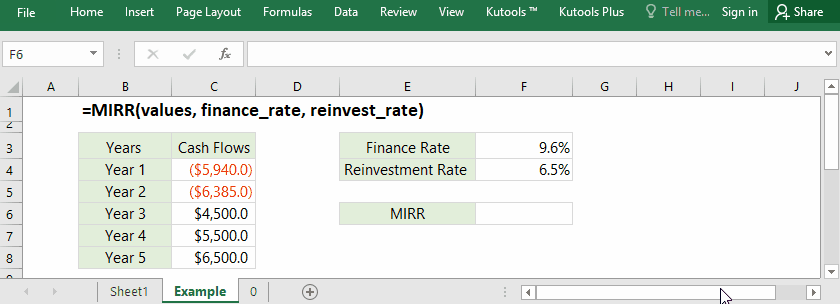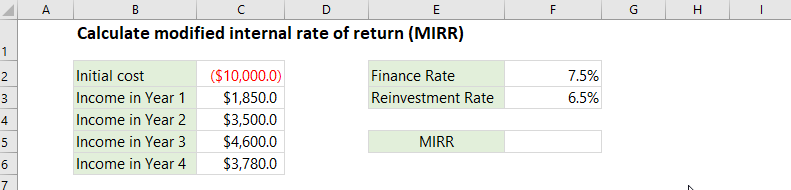Tip: Other languages are Google-Translated. You can visit the English version of this link.orRegisteror

## Excel MIRR function

We have introduced the Excel IRR function before, which can figure out the internal rate of return for a project. The IRR function assumes the interests received from the project are invested at the IRR itself. However, the positive cash flows received from the project are more likely to be reinvested at another rate. In this situation, you can use the Excel MIRR function to solve the problem.

MIRR is a modification of the IRR (internal rate of return).#### Function syntax and arguments

MIRR(values, finance_rate, reinvest_rate)

(1) Values: Required. They are the cash flow values that you will calculate the MIRR for.

It can be an array such as {-5940, -6385, 4500, 5500, 6500}, or a reference of cells such as C4:C8.
Values must consist of cash out (negative values) and cash received (positive values).

(2) Finance_rate: Required. It’s the interest rate you pay on the money used in the cash flows. It’s the cost ratio of capital.

(3) Reinvest_rate: Required. It’s the interest rate you receive on the cash flows as you reinvest them.

#### Return Value

Numerical value (percentage).

The MIRR function returns the modified internal rate of return for a series of payments and incomes occurring at regular periods.

#### Usage notes

(1) The MIRR function returns #DIV/0! error value, if the values argument contains cash out (negative numbers) only or cash received (positive numbers) only.

(2) The text, logical values, or empty cells will be ignored in the values argument; however, cells with the value zero are included.

(3) In some cases, the reinvestment rate is equal to the finance rate.

#### Formula Example: Calculate modified internal rate of return (MIRR) in Excel

Assume you invested \$10,000 initially for a project with an annual interest rate of 7.5%, and can earn money in next four years: \$1.850, \$3,500, \$4,600, and \$3,780. The reinvestment rate for the incomes is 6.5%. In this case, you can apply the MIRR function to figure out the modified internal rate of return at ease in Excel.In this case, the values are -10000,1850,3500,4600,3780 located in the Range C2:C6, the finance rate is 7.5%, the reinvestment rate is 6.5%, and you can apply one of below formulas to calculate the MIRR value:

=MIRR(C2:C6,F2,F3)

=MIRR({-10000,1850,3500,4600,3780}, 7.5%, 6.5%)

### Excel Productivity Tools

Would you like to complete your daily work quickly and perfectly? Kutools for Excel brings 300 powerful advanced features (Combine workbooks, sum by color, split cell contents, convert date, and so on...) and save 70% time.

• Designed for 1500 work scenarios, helps you solve 80% Excel problems.
• Reduce thousands of keyboard and mouse clicks every day, relieve your tired eyes and hands.
• Become an Excel expert in 3 minutes. No longer need to remember any painful formulas and VBA codes.
• 60-day unlimited free trial. 60-day money back guarantee. Free upgrade and support for 2 years.

#### Brings Efficient Tabs to Office (include Excel), Just Like Chrome, Firefox, And New IE

• Office Tab Increases your productivity by 50% when viewing and editing multiple documents.
• Reduce hundreds of mouse clicks for you every day, say goodbye to mouse hand.
• One second to switch between dozens of open documents!Say something here...
symbols left.